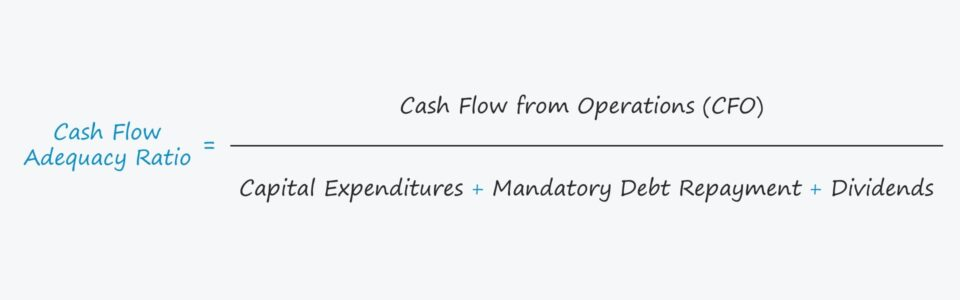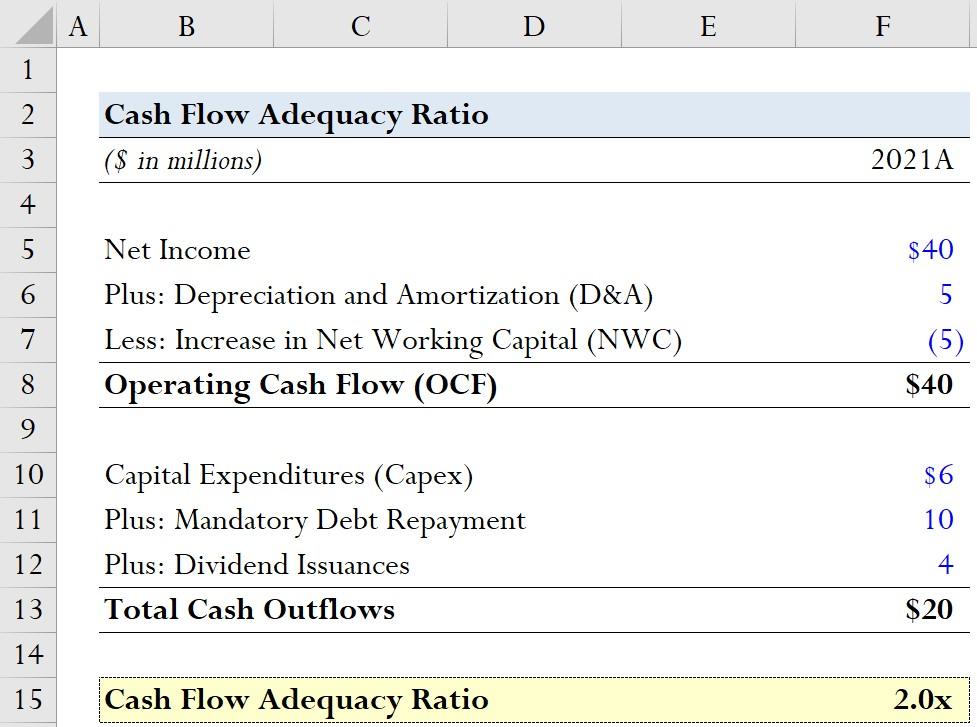Welcome to Wall Street Prep! Use code at checkout for 15% off.Guide to Understanding the Cash Flow Adequacy Ratio## How to Calculate Cash Flow Adequacy Ratio (Step-by-Step)

The cash flow adequacy ratio compares a company’s cash flows from operations to its recurring, cash outflows from investing and financing activities.

The first part of the ratio is the operating cash flow (OCF) of the company – i.e. the cash flow from operating activities section from the cash flow statement (CFS) – which represents the cash flows left over after adjustments for the following:

Operating Cash Flow (OCF) = Net Income + Depreciation & Amortization Increase in NWC

The next part of the ratio compares 1) operating cash flow (OCF) to 2) recurring, ongoing cash outflows.

More specifically, those expenditures consist of the following three items:

Technically, capital expenditures could be split into maintenance vs. growth Capex – yet, the full amount should be taken into consideration.

• Maintenance Capex → Required spending to sustain the current level of growth and continuation of operations
• Growth Capex → Discretionary spending to drive growth in excess of historical periods.

But in the case of debt, it is recommended to only include the mandatory amortization of debt principal, not the optional (early) repayment of debt.

Why? Most lenders do not necessarily expect a borrower to repay debt principal ahead of schedule.

In fact, if a borrower is underperforming, most lenders would actually prefer the company’s cash flows be allocated to maintaining the company’s operations.

This particular dynamic is a stark contrast to that of dividends paid to shareholders, which are rarely reduced once announced.

If a company decides to cut its dividend payout (or completely halt the program), the market normally assumes the worst and creates skepticism around the long-term profitability of the company, commonly resulting in a lower share price.

## Cash Flow Adequacy Ratio Formula

The formula for calculating the cash flow adequacy ratio divides the cash flows from operations (CFO) by the sum of routine capital expenditures, mandatory debt repayments and shareholder dividend issuances.

Cash Flow Adequacy Ratio = Cash Flow from Operations (CFO) ÷ (Capital Expenditures + Mandatory Debt Repayment + Dividends)

## What is a Good Cash Flow Adequacy Ratio?

The cash flow adequacy ratio can be categorized as a liquidity ratio, i.e. a metric used to assess if a company’s cash flows (or assets) are sufficient to “cover” its spending needs.

As a general rule of thumb, a higher cash flow adequacy ratio is preferable because it implies the company’s operating cash flows are adequate to cover its spending related to capex, debt repayments and dividends.

• CFA-Ratio = 1.0x → Sufficient but Limited Room for Error
• CFA-Ratio > 1.0x → Low Liquidity Risk
• CFA-Ratio < 1.0x → High Liquidity Risk

From a liquidity standpoint, the higher the ratio, the better off the company is for long-term sustainability.

On the other hand, a ratio lower than 1.0x suggests it would be in the company’s best interests to urgently seek outside funding (e.g. new equity or debt issuances), sell non-core assets (e.g. divestitures), and/or reduce total near-term spending, etc.

Like many ratios used in financial analysis, a company’s cash flow adequacy ratio can also be used to evaluate a company’s liquidity position over time to analyze ongoing trends.

Moreover, the ratio is a standardized metric, making comparisons to industry peers (i.e. direct competitors) viable.

## Cash Flow Adequacy Ratio Calculator – Excel Template

We’ll now move to a modeling exercise, which you can access by filling out the form below.Submitting...

## Cash Flow Adequacy Ratio Calculation Example

Suppose in 2021, a company generated \$40 million in net income with \$5 million in D&A and a change in NWC of \$5 million.

If we add D&A and subtract the increase in NWC to net income, we calculate the operating cash flow (OCF) as \$40 million.

• Net Income = \$40 million
• Plus: Depreciation and Amortization (D&A) = \$5 million
• Less: Increase in NWC = –\$5 million
• Operating Cash Flow (OCF) = \$40 million

In the next part of our exercise, we’ll assume the following values for our recurring cash outflows:

• Capital Expenditures (Capex) = \$6 million
• Plus: Mandatory Debt Repayment = \$10 million
• Plus: Dividend Issuances = \$4 million
• Total Cash Outflows = \$20 million

With the calculation of our two inputs complete, we can divide our hypothetical company’s operating cash flow by our aforementioned cash outflows to arrive at a cash flow adequacy ratio of 2.0x.

• Cash Flow Adequacy Ratio = \$40 million ÷ \$20 million = 2.0xStep-by-Step Online Course

### Everything You Need To Master Financial Modeling

Enroll in The Premium Package: Learn Financial Statement Modeling, DCF, M&A, LBO and Comps. The same training program used at top investment banks.Inline FeedbacksLearn Financial Modeling Online

Everything you need to master financial and valuation modeling: 3-Statement Modeling, DCF, Comps, M&A and LBO.

X

The Wall Street Prep Quicklesson Series

7 Free Financial Modeling Lessons

Get instant access to video lessons taught by experienced investment bankers. Learn financial statement modeling, DCF, M&A, LBO, Comps and Excel shortcuts.